# Dynamic Behavior

 Prev: Examples Chapter 4 - Dynamic Behavior Next: Linear Systems

In this chapter we give a broad discussion of the behavior of dynamical systems, focused on systems modeled by nonlinear differential equations. This allows us to discuss equilibrium points, stability, limit cycles and other key concepts of dynamical systems. We also introduce some methods for analyzing global behavior of solutions.

## Textbook Contents

• 1. Solving Differential Equations
• 2. Qualitative Analysis
• 3. Stability
• 4. Global Behavior
• 6. Exercises

## Chapter Summary

This chapter introduces the basic concepts and tools of dynamical systems.

1. We say that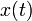is a solution of a differential equation on the time interval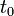to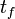with initial value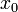if it satisfies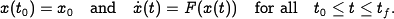We will usually assume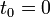. For most differential equations we will encounter, there is a unique solution for a given initial condition. Numerical tools such as MATLAB and Mathematica can be used to obtain numerical solutions forgiven the function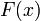.

2. An equilibrium point for a dynamical system represents a point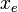such that if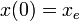then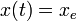for all. Equilibrium points represent stationary conditions for the dynamics of a system. A limit cycle for a dynamical system is a solutionwhich is periodic with some period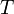, so that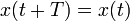for all.

3. An equilibrium point is (locally) stable if initial conditions that start near an equilibrium point stay near that equilibrium point. A equilibrium point is (locally) asymptotically stable if it is stable and, in addition, the state of the system converges to the equilibrium point as time increases. An equilibrium point is unstable if it is not stable. Similar definitions can be used to define the stability of a limit cycle.

4. Phase portraits provide a convenient way to understand the behavior of 2-dimensional dynamical systems. A phase portrait is a graphical representation of the dynamics obtained by plotting the state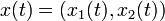in the plane. This portrait is often augmented by plotting an arrow in the plane corresponding to, which shows the rate of change of the state. The following diagrams illustrate the basic features of a dynamical systems: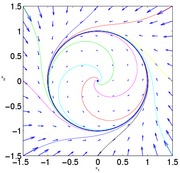An asymptotically stable equilibrium point at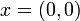. A limit cycle of radius one, with an unstable equilibrium point at. A stable equlibirum point at(nearby initial conditions stay nearby).

</p>

5. A Lyapunov function is an energy-like function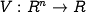that can be used to reason about the stability of an equilibrium point. We define the derivative ofalong the trajectory of the system as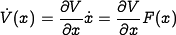Assuming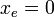and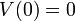, the following conditions hold:

 Condition on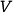Condition on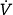Stability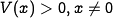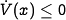for allstable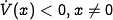asymptotically stable

Stability of limit cycles can also be studied using Lyapunov functions.

6. The Krasovskii-LaSalle Principle allows one to reason about asymptotic stability even if the time derivative ofis only negative semi-definite (rather than). Letbe a positive definite function,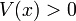for all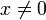and, such that

Then as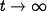, the trajectory of the system will converge to the largest invariant set inside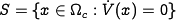.

In particular, if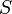contains no invariant sets other than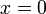, then 0 is asymptotically stable.

7. The global behavior of a nonlinear system refers to dynamics of the system far away from equilibrium points. The region of attraction of an asymptotically stable equilirium point refers to the set of all initial conditions that converge to that equilibrium point. An equilibrium point is said to be globally asymptotically stable if all initial conditions converge to that equilibrium point. Global stability can be checked by finding a Lyapunov function that is globally positive definition with time derivative globally negative definite.

</ol>